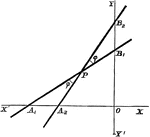### Angle

Finding the angle between two lines whose equations are in intercept form.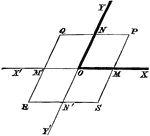### Oblique Axes

Angles on a modified coordinate plane.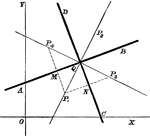### Line Bisectors

Multiple lines showing many bisectors on the coordinate plane.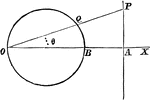### Circle

A circle and triangle situated on coordinate planes.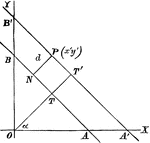### Perpendicular Distance

Finding the perpendicular distance of points whose equation is x cosa + y sina = p.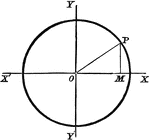### Circle Equation

Finding the equation of a circle with the origin as its center.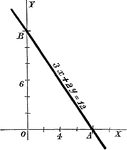### General Form

A line with a standard form equation.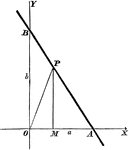### Intercept Form

A line with intercept form equation.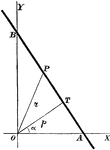### Perpendicular Form

A line on the coordinate plane showing evidence of "perpendicular" form.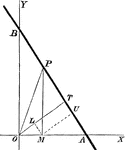### Alternate Method

Another method for using perpendicular form.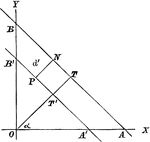### Alternate Form, Perpendicular Distance

An alternate form of the perpendicular distance method.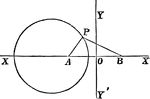### Coordinate Plane

A circle with part of a triangle inscribed in it. They are lying on a coordinate plane.